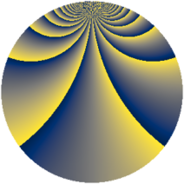# Properties

 Label 3630.2.bbLevel $3630$ Weight $2$ Character orbit 3630.bb Rep. character $\chi_{3630}(329,\cdot)$ Character field $\Q(\zeta_{22})$ Dimension $2640$ Sturm bound $1584$

# Related objects

## Defining parameters

 Level: $$N$$ $$=$$ $$3630 = 2 \cdot 3 \cdot 5 \cdot 11^{2}$$ Weight: $$k$$ $$=$$ $$2$$ Character orbit: $$[\chi]$$ $$=$$ 3630.bb (of order $$22$$ and degree $$10$$) Character conductor: $$\operatorname{cond}(\chi)$$ $$=$$ $$1815$$ Character field: $$\Q(\zeta_{22})$$ Sturm bound: $$1584$$

## Dimensions

The following table gives the dimensions of various subspaces of $$M_{2}(3630, [\chi])$$.

Total New Old
Modular forms 8000 2640 5360
Cusp forms 7840 2640 5200
Eisenstein series 160 0 160

## Trace form

 $$2640q + 264q^{4} - 8q^{9} + O(q^{10})$$ $$2640q + 264q^{4} - 8q^{9} + 22q^{10} - 264q^{16} + 20q^{25} + 8q^{34} + 8q^{36} + 48q^{45} - 136q^{49} - 88q^{51} - 28q^{55} + 264q^{64} - 36q^{66} + 16q^{69} - 4q^{70} + 32q^{75} + 88q^{79} - 56q^{81} + 200q^{91} + 56q^{99} + O(q^{100})$$

## Decomposition of $$S_{2}^{\mathrm{new}}(3630, [\chi])$$ into newform subspaces

The newforms in this space have not yet been added to the LMFDB.

## Decomposition of $$S_{2}^{\mathrm{old}}(3630, [\chi])$$ into lower level spaces

$$S_{2}^{\mathrm{old}}(3630, [\chi]) \cong$$ $$S_{2}^{\mathrm{new}}(1815, [\chi])$$$$^{\oplus 2}$$